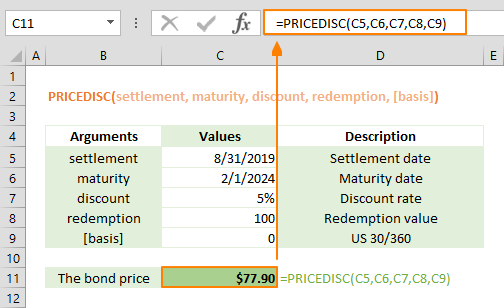The PRICEDISC Excel function is a Financial formula that calculates and returns the price per \$100 face value of a discounted security. In this guide, we’re going to show you how to use the PRICEDISC function and also go over some tips and error handling methods.

## Supported versions

• All Excel versions

## PRICEDISC Excel Function Syntax

PRICEDISC(settlement, maturity, discount, redemption, [basis])

## Arguments

 settlement The security's settlement date. The security settlement date is the date after the issue date when the security is traded to the buyer. maturity The security's maturity date. The maturity date is the date when the security expires. discount The security's discount rate. redemption The security's redemption value per \$100 face value. [basis] Optional. The type of day count basis to use. 0 or omitted: US (NASD) 30/360 1: Actual/actual 2: Actual/360 3: Actual/365 4: European 30/360

## PRICEDISC Excel Function Example

The following example shows to calculate the price per \$100 face value of a discounted security purchased on April 31st, 2019, with a maturity date of February 2nd, 2024 and a discounted rate of 5%. The redemption value is \$100, and the payments will be made based on the US (NASD) 30/360 day count.## Tips

• Microsoft recommends using the DATE or other functions which can return a date serial number.
• Excel keeps date and time values as numbers. Excel assumes that Jan 1st, 1900 is 1, and every subsequent date value is based on this. While whole numbers represent days, decimals represent time values. For example; 1/1/2018 is equal to 43101, and 12:00 is equal to 5.
• Settlement, maturity, and basis are truncated to integers.
• Other price-related functions:
• PRICE returns the price per \$100 face value of a security that pays periodic interest.
• PRICEMAT returns the price per \$100 face value of a security that pays interest at maturity.
• YIELD returns the yield on a security that pays periodic interest.
• DURATION returns the Macauley duration for an assumed par value of \$100.

## Common Issues

• If settlement or maturity are not valid dates, the PRICEDISC function returns the #VALUE! error value.
• If discount ≤ 0, PRICEDISC returns the #NUM! error value.
• The PRICEDISC returns the #NUM! error value if redemption ≤ 0.
• If [basis] < 0 or if [basis] > 4, PRICEDISC returns the #NUM! error value.
• If settlementmaturity, PRICEDISC returns the #NUM! error value.